# Workshop 6

## What Sound Is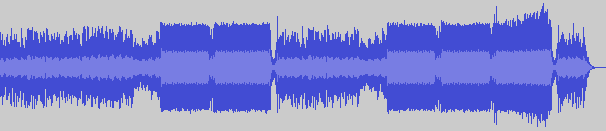"Hold Me - Luigi Talluto"

• A series of increase and decrease in air pressure, that our ears hear
• (However, the differences need to occur in a certain window of time, otherwise we don't hear it. More on that later)
• The sound we hear is a result of series of changes in values. The wider the change between one value to the next, the louder it seems; the narrower, the quieter it seems

## What Sound Is (Cont'd)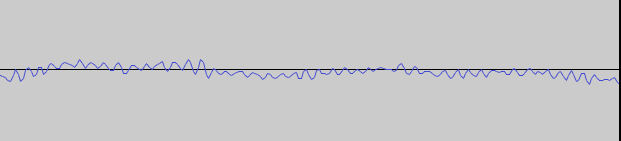The waveform from earlier but zoomed in to a particular portion of the sound. That waveform probably represents 1/500th of a second

## Loudness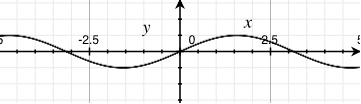amplitude: 0.3981...amplitude: 0.8912...

* Those graphs are not accurate. They just show different amplitudes

## How we perceive loudness

• humans perception of loudness is relative from one sound to the other
• the difference needs to be exponential to be perceptible

## What it takes for a person to say "hey it's louder!"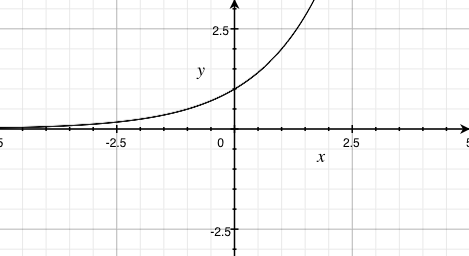## How we perceive loudness (cont'd)

• we can either represent loudness as the range of a linear function (i.e. f(x) = mx + b, where m and b are constants and x is a variable)...
• but better yet, we can represent loudness as the range of a logarithmic function (i.e. f(x) = A×logb(x/c), where A, b, and c are constants, and x is a variable)

## How we perceive loudness (cont'd)

• in signal processing, the logarithmic function used to represent "loudness" is known as the Decibel scale
• there are many Decibel scales
• Example:
• dBu: 0 dBu = 0.775 (personal stereo)
• dBV: 0 dBV = 1 (professional stage audio)
• dBFS: 0 dBFS = 1 (digital audio)

## Visually interpreting loudnesshttp://fc03.deviantart.net/fs70/f/2010/006/9/c/Equalizer_by_dl33t.gif

## Visually interpreting loudness (cont'd)

For some amplitude, A, of the given sound:

• dBu: v = 20 × log(|A|/0.775)
• dBV: v = 20 × log(|A|)
• dBFS: v = 20 × log(|A|)
• (for A ≠ 0)

## Amplifying or atenuating a signal

• A×10v/20
• Examples:
• dBu: c = 0.775×10v/20
• dBV: c = 10v/20
• dBFS: c = 10v/20

You take the resulting c and multiply it with the signal for amplification or atenuation

## Pro and Cons of using the logarithmic scale to represent loudness

• Pro
• Allows us to more easily scale loudness so that it's perceivable
• Hence why it's used on most (if not, all) devices and software
• Con
• We will never reach true silence with this scale
• Hard to even represent silence with this scale!
• why? Well, log(x) is undefined for any values of x less than or equal to 0!
• If you don't know what you're doing, a value in the Decibel scale doesn't make any sense

## The Decibel Scale (Approximation)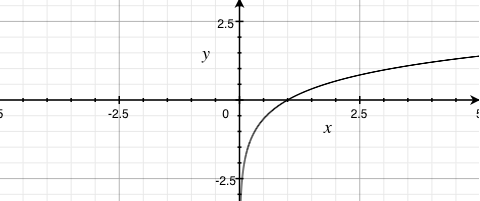## The rate of change, and pitch440 Hz, -3 dBFS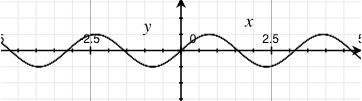880 Hz, -3 dBFS

## The rate of change, and pitch• The closer together the peaks and valleys the higher the pitch
• The farther apart, the lower the pitch

## The rate of change, and pitch

• The pitch is closely related with "frequency" (however, formally, pitch is not measured in frequency. Pitch is a measure on its own)
• The closer the peaks and valleys, the higher the frequency
• Frequency is measured in Hertz (Hz)
• Frequency determines how often a wave changes from the highest peak, to the lowest valley in a second
• So 1 Hz represents a wave going from the highest peak to the lowest valley only once in one second
• 10 Hz represents a wave going from the highest peak to the lowest valley ten times in one second

## Aside: the pitch and the human hearing

• Humans can only hear sounds as low as 16 Hz, and as high as 20,000 Hz (20 kHz)
• Any sound not within that range is never heard
• However, if loud enough, sounds below 16 Hz is often felt by the body

## Summary

• Loudness: height of peaks and valleys, also known as amplitude
• Pitch: the distance between peaks and valleys, also known as frequency

## How multiple sound sources are added together

• They're just summed together
• Three things may happen to certain parts of the wave
• Get a higher amplitude by a facter of anything greater than 1 to a maximum of 2
• Decrease in amplitude by a factor of anything in between 0 to 1 (not inclusive)
• Cancel out entirely
• So, in your applications, if you have more than one sound source, just add them up

## Application in the real world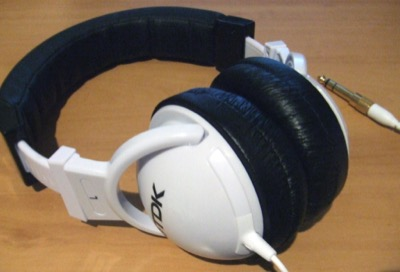Outputs the audio with amplitudes flipped

## What sound is in computer systems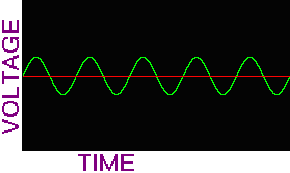http://www.alsa-project.org/alsa-doc/alsa-lib/wave1.gif A sketch of an analog waveform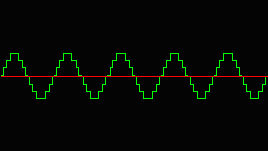http://www.alsa-project.org/alsa-doc/alsa-lib/wave2.gif A sketch of a digital waveform

## What Sound is in computer systems (cont'd)

• when it comes to DSP, computer systems don't work with continuous streams
• They work with finite and discrete set of ordered numbers (e.g. numbers stored in arrays)
• These sets are formally called point-code modulation (PCM)
• Elements inside PCM are called "samples"

## What Sound is in computer systems (cont'd)

• in modern day computers, one second of audio is popularly encoded in 44100 samples
• However, a second of audio can be encoded in less or more samples, if preferred
• That 44100 samples per second number is more formally called the "samplerate"
• The unit for samplerate is Hertz (Hz)
• As far as samples is concerned, Hertz is the unit of measure of how many things happen in a second
• (If some audio data has a samplerate of x, how long does a single sample last?)

## What sound is in computer systems (cont'd)

• to mimic audio localization, more than one audio sources can be recorded and played back
• when more than one set of PCM data is available for playback, these sets are called "channels"
• commonly, most digital audio sources are encoded to have two channels
• one to be played back by a "left" speaker
• the other to be played by a "right" speaker
• some can have five channels (such as Dolby Surround sound): one for front-left, front-right, back-left, back-right, and centre

## What Sound is in computer systems (cont'd)

• PCM data can be stored as raw data (WAV)
• In a—for example—WAV file, if more than one PCM data set is available for more channels, the header data must indicate that there is more than one set of data available
• PCM data can be stored losslessly compressed (ALAC, FLAC)
• Again, headers must indicate the number of PCM data that is available
• PCM data can also be lossily compressed (Ogg Vorbis, AAC, MP3)
• Again, headers must indicate the number of PCM data that is available

## What sound is in computer systems (cont'd)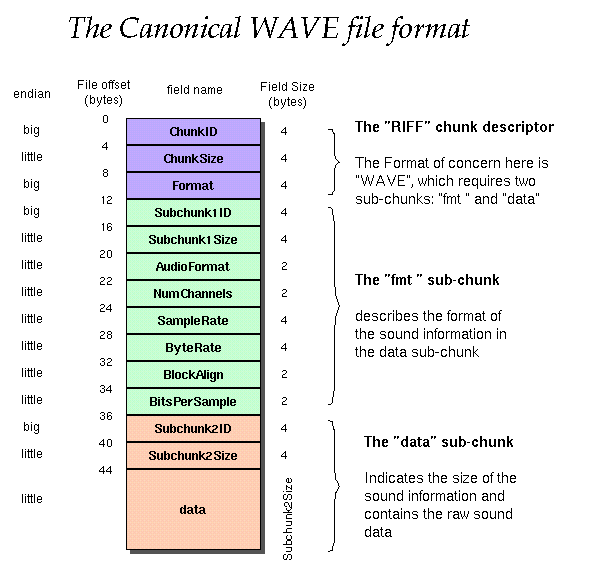https://ccrma.stanford.edu/courses/422/projects/WaveFormat/wav-sound-format.gif

## What sound is in computer systems (cont'd)

• Although, not always, but some software systems prefer working with powers of 2 chunks of PCM data at the time
• Allows for better analysis (spectral analysis) of PCM data
• The larger these chunks, the better our analysis and modification, however, it is much slower to parse
• The smaller these chunks, the worse our analysis and modification, however, it is much faster to parse

## What sound is in computer systems (cont'd)

An example in JavaScript

``````
var context = new AudioContext();
var processor = context.createScriptProcessor(
// Chunk (buffer) size.
512,
// Number of input channels
1,
// Number of output channels
2
);
processor.onaudioprocess = function (e) {
var L = e.outputBuffer.getChannelData(0);
var R = e.outputBuffer.getChannelData(1);
// L and R are both mutable arrays of numbers. Do stuff with them.
}
``````

## What Sound is (A Theoretical approach)

Fourier's theorem: a wave form is the sum of many sine waves, each with different periods (a.k.a. frequencies), phases and amplitudes

These sine waves are also often called "harmonics"

## Review: A Sine Wave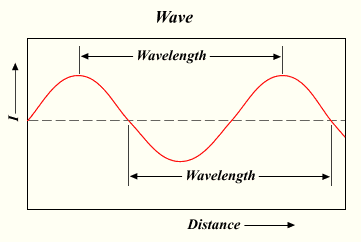• The sine wave is generated by the sine function (often abbreviated to `sin`)
• drawing the sine wave on a graph is simply
`for (var x = 0; x < someLargeValue; x++) { y = sin(x); }`

## Review: A Sine Wave (cont'd)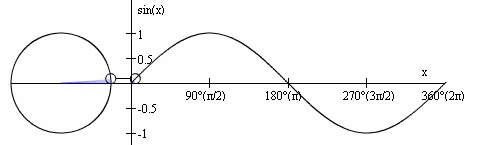http://media.giphy.com/media/NKLdcqhwo2f8A/giphy.gif

• The sine function's input (domain) is a rotation value, which we normalize to 2π
• The sine function's output (range) represents the vertical (often called y) coordinate when drawing a circle at a particular angle (where that circle's centre is located at the origin (0,0))

## Review: A Sine Wave (cont'd)sin(x)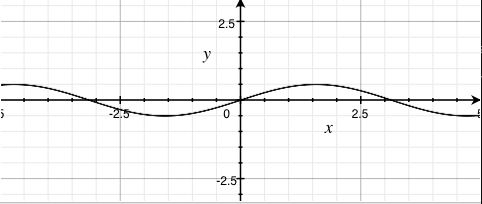0.5sin(x)3sin(x)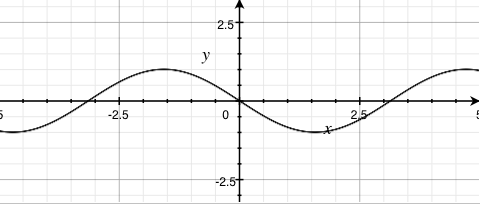-sin(x)
• Increasing the coefficient (greater than 1) on the sine function's output results in results in larger amplitudes
• Clamping the coefficient to anywhere between 0 and 1 (not inclusive) on the sine function's output results in smaller amplitudes
• Negating the output results in an inverted output

## Review: A Sine Wave (cont'd)sin(x)sin(2x)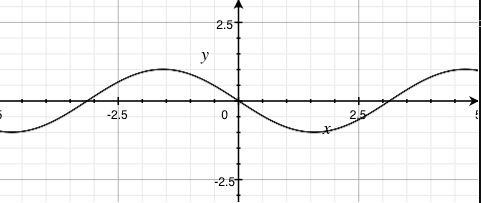sin(-x)
• Increasing the coefficient on the sine function's input results in shorter periods (higher frequencies)
• Clamping the coefficient to anywhere between 0 and 1 (not inclusive) on the sine function's input results in longer periods (lower frequencies)
• Negating the output result in the output going the other way

## Review: A Sine Wave (cont'd)sin(x)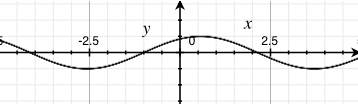sin(x - 1)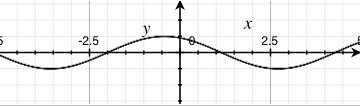sin(x + 1)
• adding by a constant results in a translation of the sine wave by that constant's amount towards the left
• conversely, subtracting by a constant results in a translation of the sine wave by that constant's amount towards the right

## A simple square wave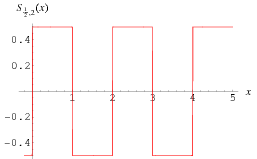http://mathworld.wolfram.com/images/eps-gif/SquareWave_700.gif

## A simple square wave (cont'd)

Recall, fourier's theorem: a wave is a sum of its sine waves

http://i.stack.imgur.com/6yngK.gif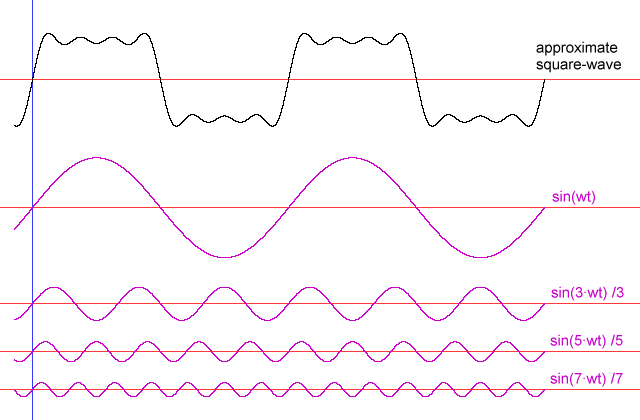## A simple square wave (cont'd)

http://i.stack.imgur.com/JhZnq.png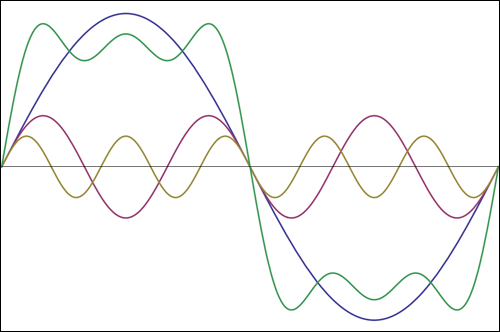Note: the green line represents the sum of the waves. All others represents the harmonics

## A simple square wave (cont'd)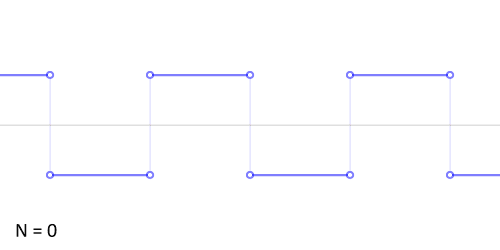## Another example: the sawtooth wave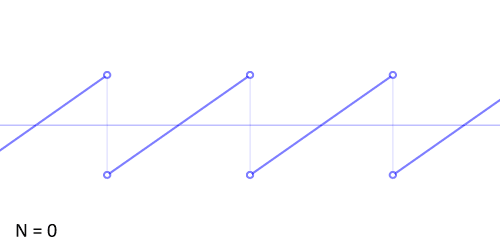## Digitally Extracting Harmonics from waves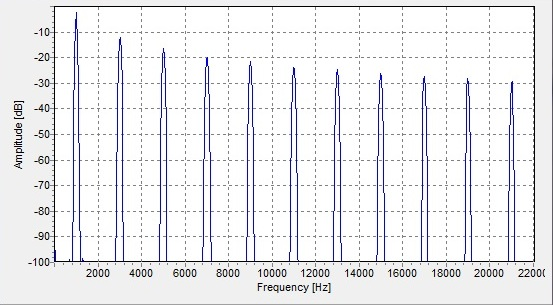## Extracting Harmonics from waves

Discrete Fourier Transform (DFT)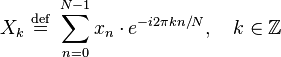http://nayuki.eigenstate.org/res/how-to-implement-the-discrete-fourier-transform/dft.js

``````
var n = pcm.length;
var out = new Array(n);
for (var k = 0; k < n; k++) {  // For each output element
var sumreal = 0;
var sumimag = 0;
for (var t = 0; t < n; t++) {  // For each input element
var angle = 2 * Math.PI * t * k / n;
sumreal += inreal[t] * Math.cos(angle);
sumimag += inreal[t] * Math.sin(angle);
}
out[k] = {
real: sumreal,
imaginary: sumimag
};
}
``````

The above algorithm is a proof of concept only. It should never be used for real-time DSP! (For anything that isn't real-time is fine.) Use a library that implements a fast Fourier transform (FFT), instead

## Extracting Harmonics (cont'd)

• the DFT outputs a set of complex numbers
• each element of the set encodes two values
• the amplitude
• the phase
• Don't be fooled by the JavaScript code. Although each element of the `out` array has two properties, they're actually related. They're in two properties because JavaScript lacks a "complex number" primitive

## Extracting the amplitude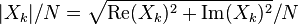``````
var amps = new Array(n);
for (var k = 0; k < n; k++) {
var real = out[k].real;
var imaginary = out[k].imaginary;
amps[k] = Math.sqrt(real*real + imaginary*imaginary)/n;
}
``````

## Extracting the phases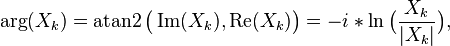``````
var phases = new Array(n);
for (var k = 0; k < n; k++) {
phases[k] = Math.atan2(out[k].imaginary, out[k].real);
}
``````

## Reconstructing the original wave (almost)

``````
var pcm = new Array(n);
for (var k = 0; k < n; k++) {
pcm[k] = 0;
for (var j = 0; j < n; j++) {
pcm[k] += Math.sin(2 * Math.PI * k/sampleRate + phases[j]) * amps[j];
}
}
``````

Never use the above algorithm in any real-time DSP applications!

## Applications of DFT (and FFT)

• changing pitch of certain sounds
• extract specific features of audio, such as voice or instrumental
• extract melodies of leads of musical tracks
• applying effects to electronic music
• mimicking localization on stereo audio
• extracting spectral information for equalizer information
• studying ambient noise

## Applying everything (Web Audio API)

``````
var SAMPLES_BUFFER_SIZE = 128; // Can be any powers of 2.
var buffer = new Uint8Array(SAMPLES_BUFFER_SIZE);

var audioContext = new AudioContext();

var fft = audioContext.createAnalyser();
fft.fftSize = SAMPLES_BUFFER_SIZE;

// We'll initialize some audio source, and call it `src`

// There you have it, we are now performing frequency analysis on the audio.
src.connect(fft);

fft.getByteFrequencyData(buffer);

// `buffer` should now be populated with frequency domain data, with the ranges
// representing amplitudes in a logarithmic scale.
``````